# Digital Predistortion to Compensate for Power Amplifier Nonlinearities

This demo shows an example of using digital predistortion (DPD) in a transmitter to offset the effects of nonlinearities in a power amplifier. In this case, we use a model of a power amplifier that was obtained using the Power Amplifier Characterization (Communications Toolbox). In the first simulation, the RF transmitter sends two tones. In the second simulation, the RF transmitter sends a 5G-like OFDM waveform with 100 MHz bandwidth.

### DPD with Two Sinusoidal Test Signals

Open the Simulink RF Blockset model including the PA model and the adaptive DPD algorithm [ 2 ]:

System-level model PA + DPD with two tones

The model includes a two-tone signal generator that is used for testing the output-referred third-order intercept point of the system. The model includes upconversion to RF frequency using an I-Q modulator, the PA model, a coupler to sniff the output of the PA, and an S-parameter block representing the antenna loading effect. The receiver chain performs downconversion to low intermediate frequency. Notice that the simulation bandwidth of this system is 107.52 MHz.

The model can be simulated without the DPD when the toggle switch is in the up position.

```model = 'simrfV2_powamp_dpd'; open_system(model) sim(model) ```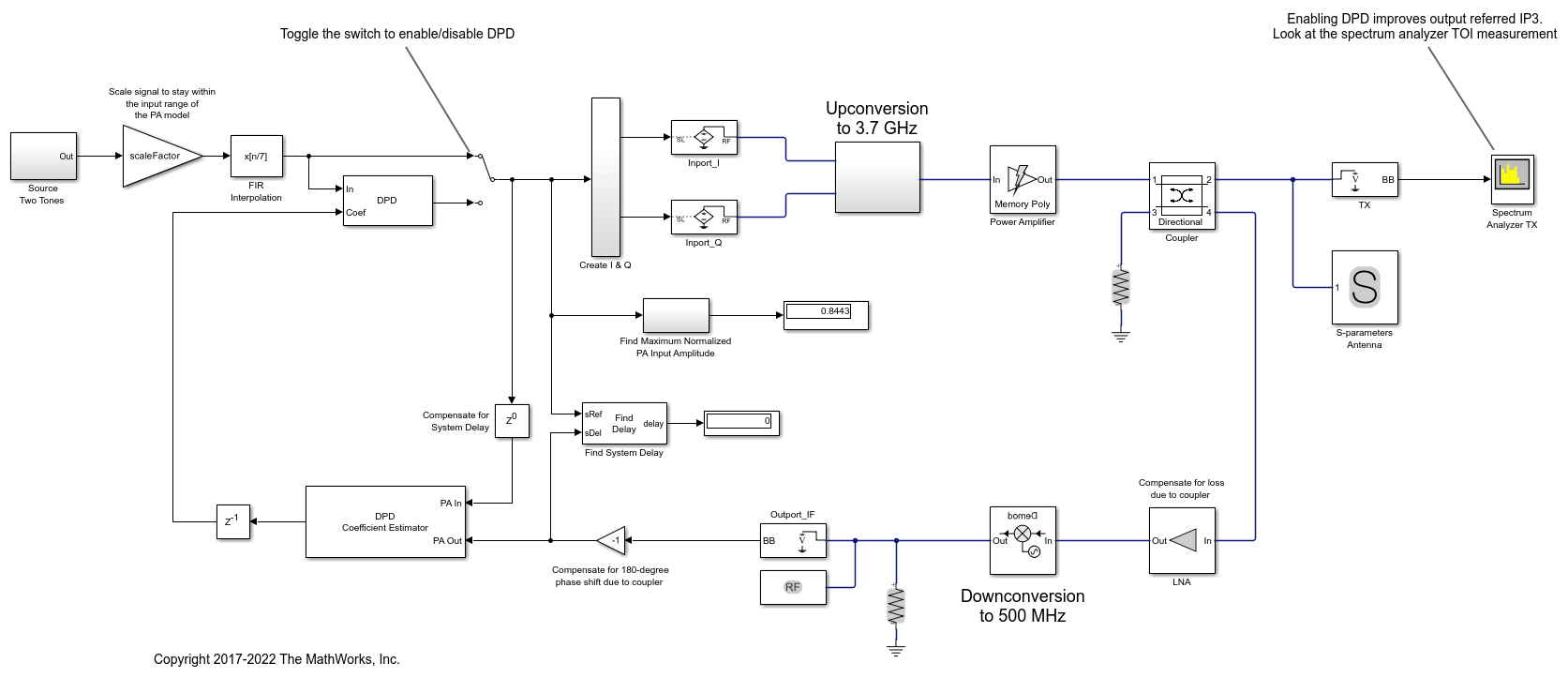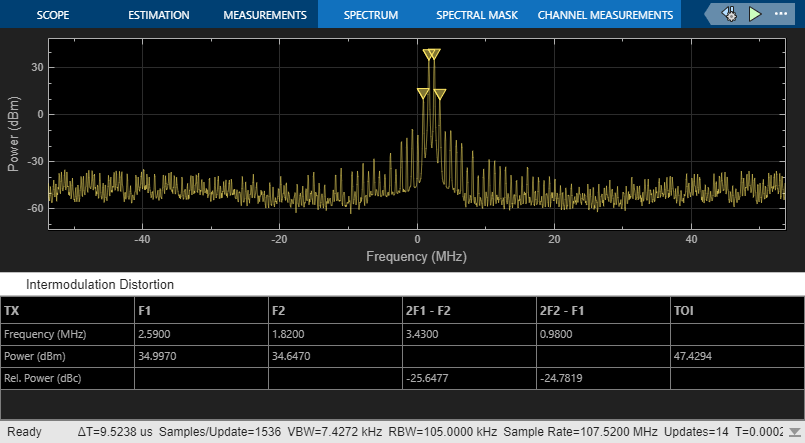The manual switch is toggled to enable the DPD algorithm. When toggled, the TOI (third-order intercept point) is improved by more than 10 dB within 0.2 ms of simulation time. Inspect the distortion measurement in the Spectrum Analyzer to validate these results and see how the power of the harmonics is reduced thanks to the DPD linearization.

```set_param([model '/Manual Switch'], 'action', '1') sim(model) ```By changing the degree and the memory depth defined in the DPD Coefficient Estimator block, you can find the most suitable tradeoff between performance and implementation cost.

To estimate the DPD coefficients correctly, the input signals to the DPD Coefficient Estimator block, PA In and PA Out, must be aligned in the time domain. This is verified by the Find Delay block which shows that the delay introduced by the RF system is 0. The estimator's desired amplitude gain is important to make sure that the total linear gain of the system does not change when the DPD is active. For this particular model, the gain has been estimated using separate techniques [ 2 ].

```close_system(model,0) close all; clear ```

### DPD with a 5G-like OFDM Waveform

Open the Simulink RF Blockset model including the PA model and the adaptive DPD algorithm excited with a 5G-like OFDM waveform with 100 MHz bandwidth.

System-level model PA + DPD with a 5G-like OFDM waveform

Without DPD linearization, the system has a Modulation Error Ratio of 26 dB, as it can be seen from the constellation plot measurement.

```model = 'simrfV2_powamp_dpd_comms'; open_system(model) sim(model) ```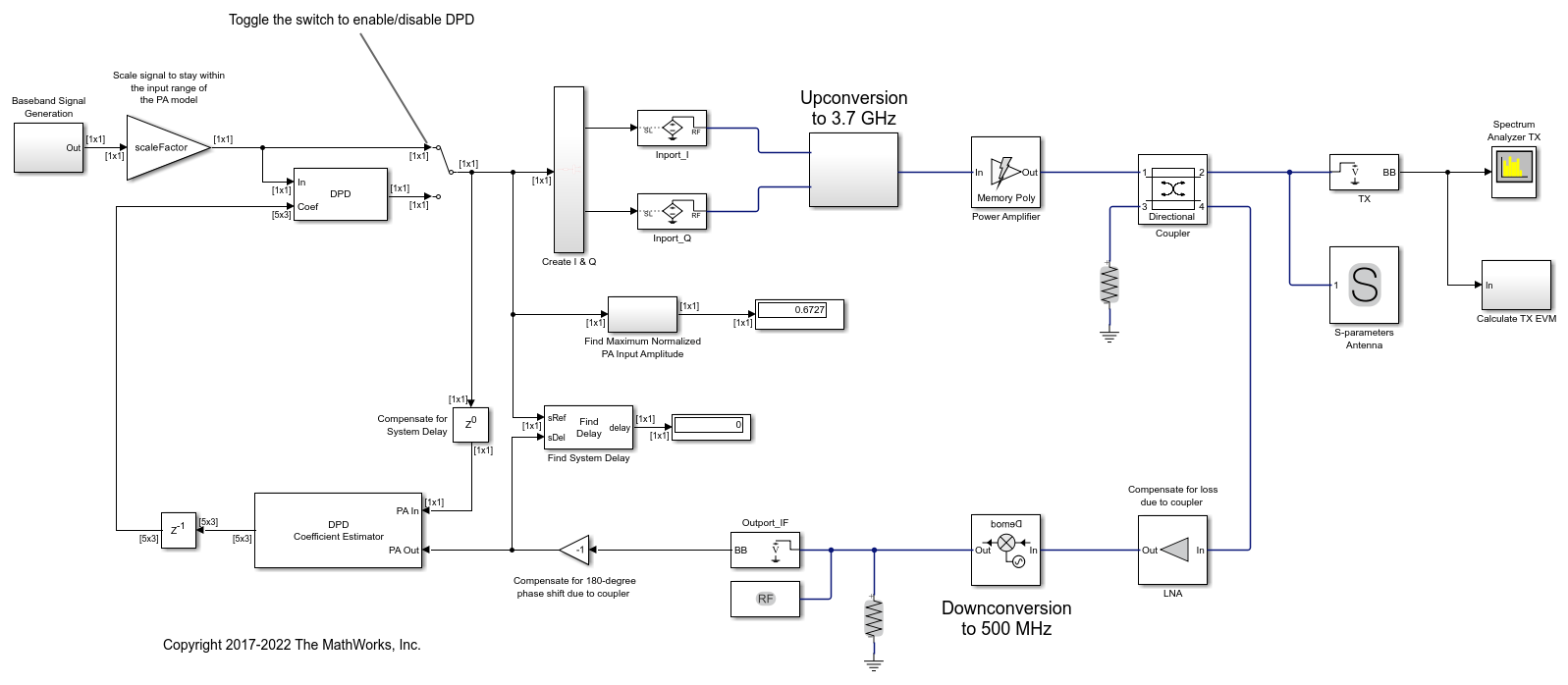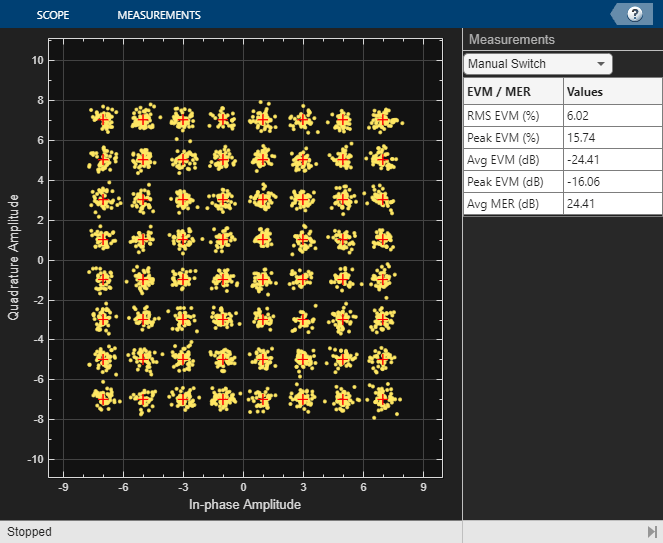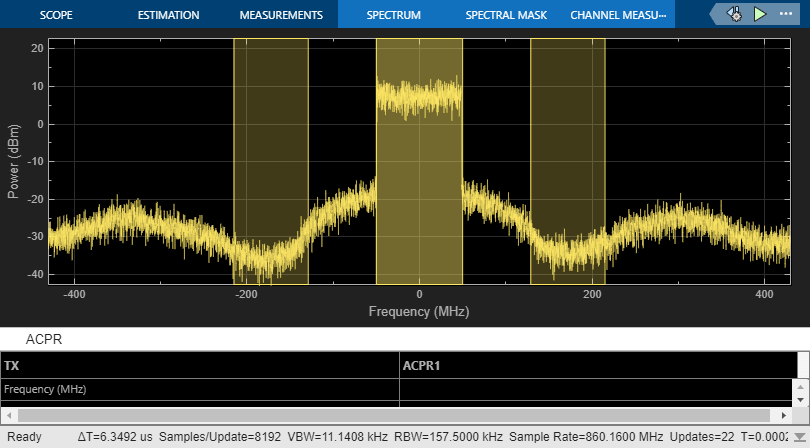The manual switch is toggled to enable the DPD algorithm. When toggled, the average MER is improved from 26 dB to approximately 33 dB in about 0.1 ms of simulation time.

```set_param([model '/Manual Switch'], 'action', '1') sim(model) ```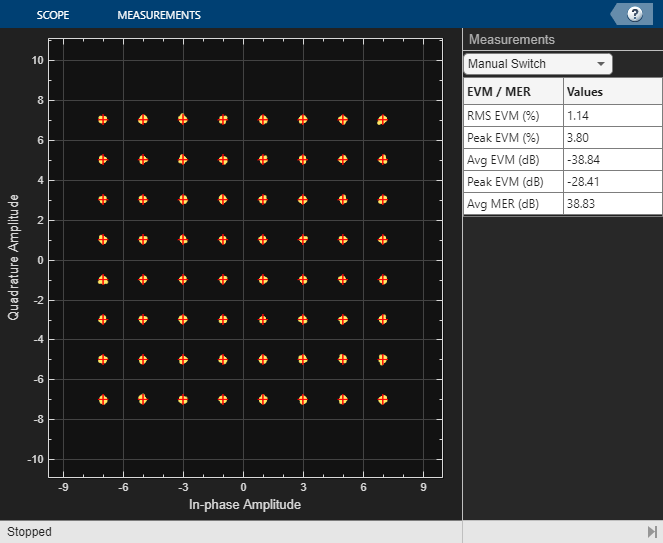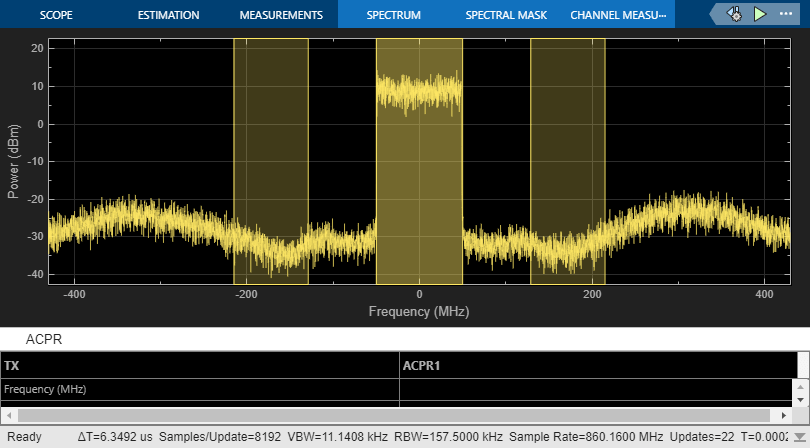```close_system(model,0) close all; clear ```

### Selected Bibliography

1. Morgan, Dennis R., Zhengxiang Ma, Jaehyeong Kim, Michael G. Zierdt, and John Pastalan. "A Generalized Memory Polynomial Model for Digital Predistortion of Power Amplifiers." IEEE® Transactions on Signal Processing. Vol. 54, No. 10, October 2006, pp. 3852–3860.

2. Gan, Li, and Emad Abd-Elrady. "Digital Predistortion of Memory Polynomial Systems Using Direct and Indirect Learning Architectures." In Proceedings of the Eleventh IASTED International Conference on Signal and Image Processing (SIP) (F. Cruz-Roldán and N. B. Smith, eds.), No. 654-802. Calgary, AB: ACTA Press, 2009.